1594641600

Some facts just mess up in our minds and then it gets hard to recall what’s what. I had a similar experience with Bias & Variance, in terms of recalling the difference between the two. And the fact that you are here suggests that you too are muddled by the terms.

So let’s understand what Bias and Variance are, what Bias-Variance Trade-off is, and how they play an inevitable role in Machine Learning.

## The Bias

Let me ask you a question. Why do humans get biased when they do? Or what motivates them to show some bias every now and then?

I’m sure you had a good answer or many good answers. But to summarise them all, the most fundamental reason that we see bias around us is —_ ease of mind._

Being humans, it’s easy to incline our thoughts and favors towards something we like, we admire, or something we think is right, without bending our thoughts much.

For most of our life’s decisions, we don’t want to put our brains into analyzing each and every scenario. Now one might be investigative, meticulous, or quite systematic while doing things that are important and consequential, but for the most part, we are too lazy to do that.

But how this human intuition of being biased is related to Machine Learning? Let’s understand how.

Consider the figure below.

One could easily guess that this figure represents Simple Linear Regression, which is an _inflexible _model that assumes a linear relationship between input and output variables. This assumption, approximation, and restriction introduce _bias _to this model.

Hence bias refers to the error which is observed while approximating a complex problem using a simple (or restrictive) model.

This analogy between humans and machines could be a great way to understand that inflexibility brings bias.

Observe the figure below. The plots represent two different models that were used to fit the same data. Which one do you think will result in higher bias?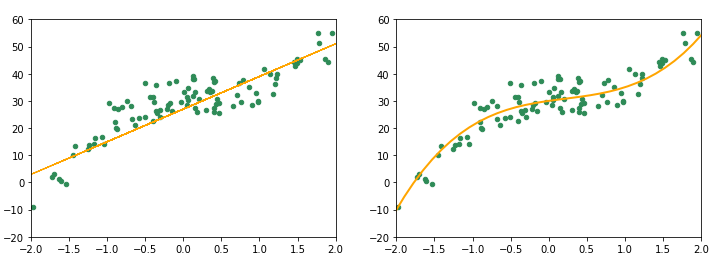The plot on the right is quite more flexible than the one on the left. It fits more smoothly with the data. On the other hand, the plot on the left represents a poorly fitted model, which assumes a linear relationship in data. This poor-fitting due to high bias is also known as underfitting. Underfitting results in poor performance and low accuracies and can be rectified if needed by using more flexible models.

Let’s summarise the key points about bias:

• Bias is introduced when restrictive (inflexible) models are used to solve complex problems
• As the flexibility of a model increases, the bias starts decreasing for training data.
• Bias can cause underfitting, which further leads to poor performance and predictions.

So how can we get rid of this bias? We can build a more flexible model to fit our data and remove underfitting.

So should we keep building more complex models until we reduce the error to its minimum? Let’s try and do that with some randomly generated data.

#machine-learning #bias #variance #data-science #data analysis

## Buddha Community1603004400

In the process of building a Predictive Machine learning model, we come across the Bias and Variance errors. The Bias-Variance Tradeoff is one of the most popular tradeoffs in Machine Learning. Here, we will go over what Bias error and Variance error are, sources of these errors and how you can work to reduce these errors in your model.

How does Machine Learning differ from traditional programming?

The high school definition of a program was simple. A program is a set of rules that tells the computer what to do and how to do it. This is one of the main difference between traditional programming and Machine Learning.

In traditional programming, the programmer defines the rules. The rules are usually well defined and a programmer often has to spend a good amount of time debugging code to ensure that the code runs smoothly.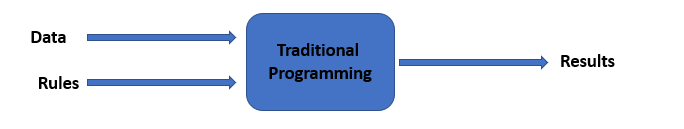In Machine Learning, while we still we still code, we do not define the rules. We build a model and feed it our expected results (supervised ML) or we allow the model come up with its own results (unsupervised ML). The main focus in Machine Learning is to improve the accuracy of the initial guess the model makes.1594560000

## Bias Variance decomposition

We often wonder how to select a method from a pool of machine learning methods which gives best results for a given dataset.

“The process of selecting the best model with appropriate complexity for a specific problem statement is known as model selection”

This brings us to a very important property of statistical learning methods known as bias variance trade off, that emphasizes on how well a model is learning the associations in the training datasets.

In this article, we will discuss what is bias and variance, and how to reduce them. Finally, we will implement a few practical illustrations to see how these concepts can be applied in model building.

So, let’s get started.

There are 3 types of error in predictions due to deviation from the actual truth:

1. Irreducible error
2. Bias
3. Variance

Irreducible error is a measure of noise inherent in the data. There might always be some predictors which have some small effect on the target variable and are not part of our model.

Thus, no matter how good a model we might select, it will not be able to approximate the actual function perfectly, hence leaving the error which cannot be reduced.

So, let’s focus on what we can control while building model i.e. bias and variance and how.

For this purpose, we will use ‘mlxtend’ library (developed by Sebastian Raschka) to calculate ‘bias_variance_decomp’ .1598278920

Supervised Learning can be best understood by the help of Bias-Variance trade-off. The main aim of any model comes under Supervised learning is to estimate the target functions to predict the output with the help of input variables. Supervised learning consists of the Machine learning Algorithms, that are used for the data for its analysis by looking at its previous outcomes. Every action, has its outcomes or final target which helps it to be useful. Supervised Learning takes the help of the actions and its previous outcomes to analyze it and predict the possible outcomes of future. In Supervised Learning every algorithms function on some previous known data which is labeled; labeled here means that every information about the data is given. Algorithms is being trained on that labelled data repeatedly and then machine performs the actions based on that training to predict the outcomes. These predicted outcomes are more or less very similar to the past outcomes. This helps us to take decisions for the actions that hasn’t been occurred yet. Whether it is weather forecasting, predicting stock market price, house/property price, detecting email spam, recommendation system, self-driving car, churn modelling, sale of products etc., Supervised Learning comes into actions. In Supervised Learning, you supervise the learning process, meaning the data that you have collected here is labelled and so you know what input needs to be mapped to what output. it is the process of making an algorithm to learn to map an input to a particular output. This is achieved using the labelled datasets that you have collected. If the mapping is correct, the algorithm has successfully learned. Else, you make the necessary changes to the algorithm so that it can learn correctly. Supervised Learning algorithms can help make predictions for new unseen data that we obtain later in the future. It is as same as the teacher-student scenario. A teacher teaches the students to learn from the book (labelled datasets), and students learn from it and later on gives the test (prediction of algorithm) to pass. If the student fails (overfitting or underfitting), teacher tune the students (hyperparameter tuning) to perform better later on. But theirs a lot to catch-up between what is an ideal condition and what in practical possible. As no students (Algorithms) or teacher (datasets) can be 100 percent true or correct in their work. Same way, there are many advantages and disadvantages of every model and data that is been feeded into the model. Datasets might be unbalanced, consists of many missing values, improperly shaped and sized, can contains many outliers that makes any model task difficult to perform. Similarly, every model has its disadvantages or makes error in mapping the outputs. I will talk about these errors that prevent models to perform best and how can we overcome those errors.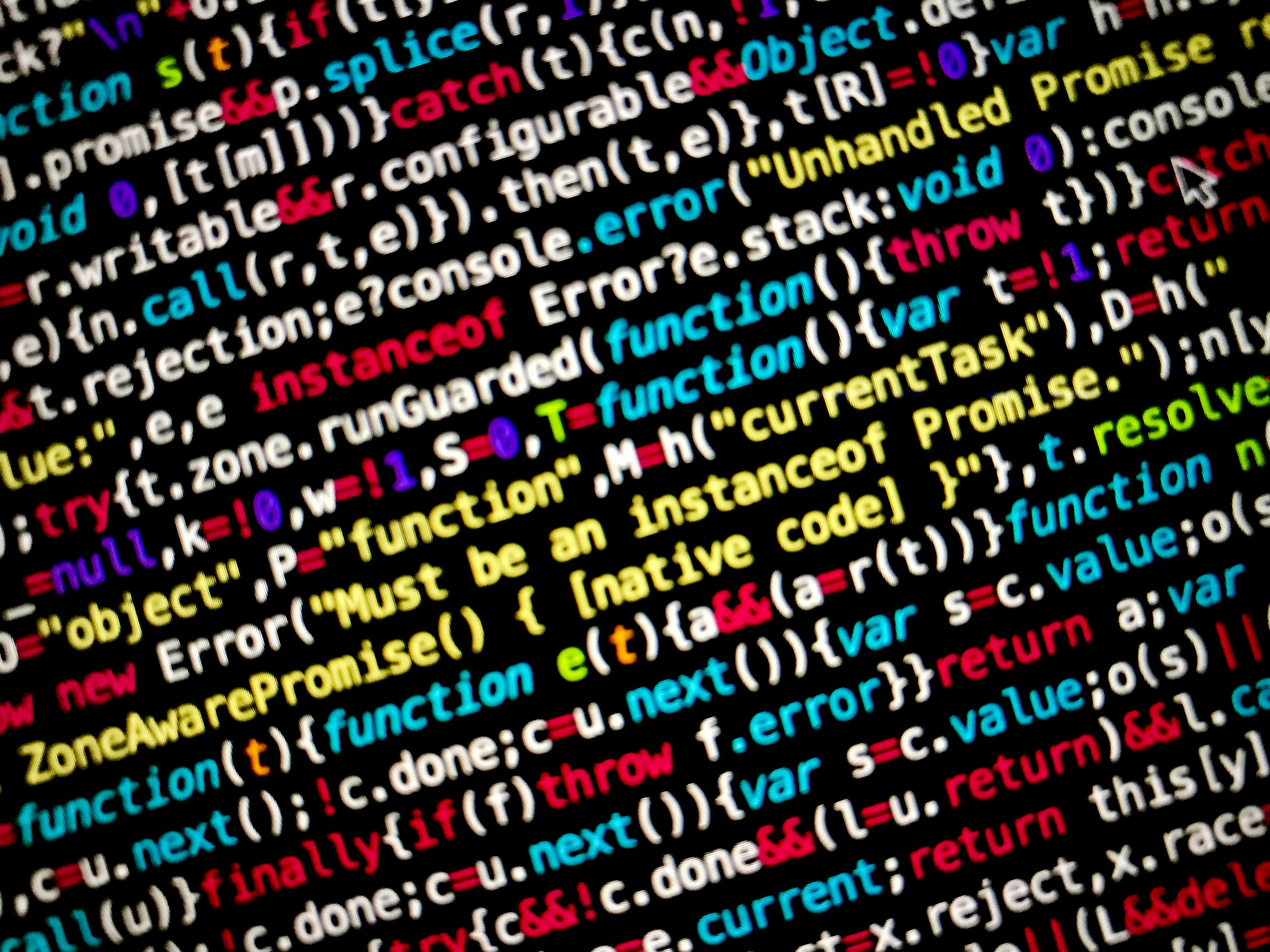Photo by Max Chen on Unsplash

Before proceeding with the model training, we should know about the errors (bias and variance) related to it. If we know about it, not only it would help us with better model training but also, helps us to deal with underfitting and overfitting of model.

This predictive error is of three types:

1. Bias

2. Variance

3. Irreducible error1597020720

## Bias and Variance: High Bias,High Variance

If you run a learning algorithm and it doesn’t perform good as you are hoping, it will be because you have either a high bias problem or a high variance problem, in other words, either an underfitting problem or an** overfitting** problem almost all the time.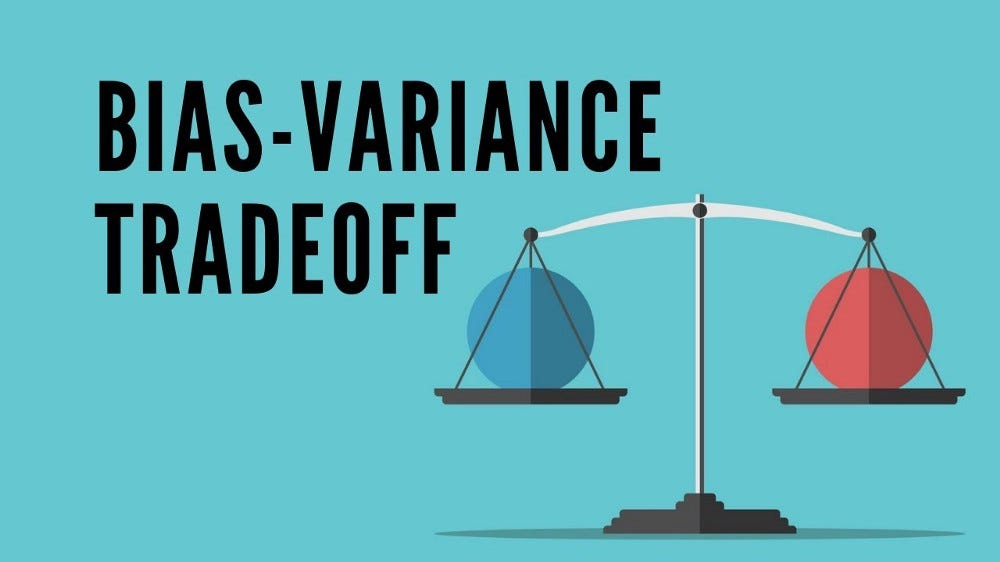It is vital to understand which of these two problems is bias or variance or a bit of both because knowing which of these two things is happening would give a very strong indicator for promising ways to try to improve the algorithm.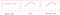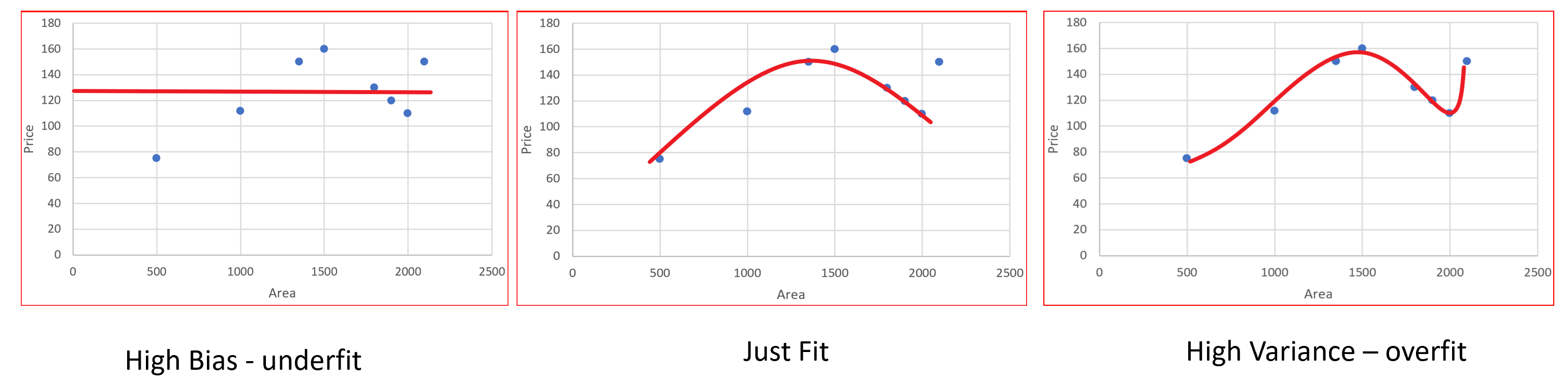### High Bias :

• The graph has an overly low order polynomial to fit the data. The hypothesis will not the fit the training set well, we need a higher degree polynomial to fit the data in this case.
• The training set error and cross validation error both are going to be high.
• Cross validation error will close to or slightly higher than training set.
• This is the case of underfitting.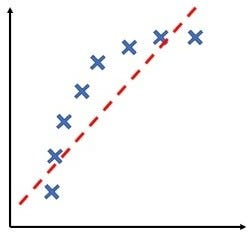Underfitting

If these are the signs then your algorithm might be suffering from high bias.

### High Variance :

• The graph has too large degree of polynomial to fit the data. The hypothesis will not fit the training set well, we need a bit lower degree polynomial to fit the data in this case.
• The data will be fitting the training set very well i.e. the training set error will usually be low but the error of cross-validation set will be much larger than the training error.
• This is the case of overfitting.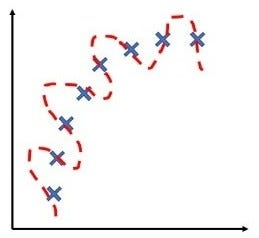Overfitting

If these are the signs then your algorithm might be suffering from high variance.

#variance #machine-learning #bias #overfitting #underfitting #deep learning1594641600

Some facts just mess up in our minds and then it gets hard to recall what’s what. I had a similar experience with Bias & Variance, in terms of recalling the difference between the two. And the fact that you are here suggests that you too are muddled by the terms.

So let’s understand what Bias and Variance are, what Bias-Variance Trade-off is, and how they play an inevitable role in Machine Learning.

## The Bias

Let me ask you a question. Why do humans get biased when they do? Or what motivates them to show some bias every now and then?

I’m sure you had a good answer or many good answers. But to summarise them all, the most fundamental reason that we see bias around us is —_ ease of mind._

Being humans, it’s easy to incline our thoughts and favors towards something we like, we admire, or something we think is right, without bending our thoughts much.

For most of our life’s decisions, we don’t want to put our brains into analyzing each and every scenario. Now one might be investigative, meticulous, or quite systematic while doing things that are important and consequential, but for the most part, we are too lazy to do that.

But how this human intuition of being biased is related to Machine Learning? Let’s understand how.

Consider the figure below.

One could easily guess that this figure represents Simple Linear Regression, which is an _inflexible _model that assumes a linear relationship between input and output variables. This assumption, approximation, and restriction introduce _bias _to this model.

Hence bias refers to the error which is observed while approximating a complex problem using a simple (or restrictive) model.

This analogy between humans and machines could be a great way to understand that inflexibility brings bias.

Observe the figure below. The plots represent two different models that were used to fit the same data. Which one do you think will result in higher bias?The plot on the right is quite more flexible than the one on the left. It fits more smoothly with the data. On the other hand, the plot on the left represents a poorly fitted model, which assumes a linear relationship in data. This poor-fitting due to high bias is also known as underfitting. Underfitting results in poor performance and low accuracies and can be rectified if needed by using more flexible models.

Let’s summarise the key points about bias:

• Bias is introduced when restrictive (inflexible) models are used to solve complex problems
• As the flexibility of a model increases, the bias starts decreasing for training data.
• Bias can cause underfitting, which further leads to poor performance and predictions.

So how can we get rid of this bias? We can build a more flexible model to fit our data and remove underfitting.

So should we keep building more complex models until we reduce the error to its minimum? Let’s try and do that with some randomly generated data.

#machine-learning #bias #variance #data-science #data analysis